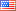# Revision history [back]

### dual of weyl group

I was wondering if, given an element in a Weyl group, to produce the corresponding element in the dual Weyl group. As an example, if w in W = RootSystem(['A', 3]).weight_lattice().weyl_group()

Then I would like a function f such that f(w) in RootSystem(['A', 3]).coroot_lattice().weyl_group(), with the obvious duality <w*x,y> = <x,f(w)*(y)&gt;, where="" x="" in="" the="" weight="" lattice="" and="" y="" is="" in="" the="" coroot="" lattice.<="" p="">

thanks2 formattingMike Hansen4058 ●24 ●48 ●89

### dual of weyl group

I was wondering if, given an element in a Weyl group, to produce the corresponding element in the dual Weyl group. As an example, if w w in W = RootSystem(['A', 3]).weight_lattice().weyl_group()

Then I would like a function f such that f(w) f(w) in RootSystem(['A', 3]).coroot_lattice().weyl_group(), 3]).coroot_lattice().weyl_group(), with the obvious duality <w*x,y> = <x,f(w)*(y)&gt;, where="" x="" in="" the="" weight="" lattice="" and="" y="" is="" in="" the="" coroot="" lattice.<="" p=""> <x,f(w)*(y)>, where x in the weight lattice and y is in the coroot lattice.

thanks

### dual of weyl group

I was wondering if, if it is possible to, given an element in a Weyl group, to produce the corresponding element in the dual Weyl group. As an example, if w in W = RootSystem(['A', 3]).weight_lattice().weyl_group()

Then I would like a function f such that f(w) in RootSystem(['A', 3]).coroot_lattice().weyl_group(), with the obvious duality <w*x,y> = <x,f(w)*(y)>, where x in the weight lattice and y is in the coroot lattice.

thanks4 retaggedFrédéricC4383 ●3 ●37 ●93

### dual of weyl group

I was wondering if it is possible to, given an element in a Weyl group, produce the corresponding element in the dual Weyl group. As an example, if w in W = RootSystem(['A', 3]).weight_lattice().weyl_group()

Then I would like a function f such that f(w) in RootSystem(['A', 3]).coroot_lattice().weyl_group(), with the obvious duality <w*x,y> = <x,f(w)*(y)>, where x in the weight lattice and y is in the coroot lattice.

thanks5 retaggedFrédéricC4383 ●3 ●37 ●93

### dual of weyl group

I was wondering if it is possible to, given an element in a Weyl group, produce the corresponding element in the dual Weyl group. As an example, if w in W = RootSystem(['A', 3]).weight_lattice().weyl_group()

Then I would like a function f such that f(w) in RootSystem(['A', 3]).coroot_lattice().weyl_group(), with the obvious duality <w*x,y> = <x,f(w)*(y)>, where x in the weight lattice and y is in the coroot lattice.

thanks